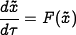# Difference between revisions of "Errata: In Exercise 4.1, x 0 should not be subtracted from time-shifted solution"

The printed version of the problem incorrectly shifts the initial condition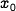. The corrected version of the problem should read:
Show that if we have a solution of the differential equation (4.x) given by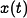with initial condition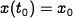, then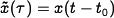is a solution of the differential equation